Support the Monkey! Tell All your Friends and TeachersHome MonkeyNotes Printable Notes Digital Library Study Guides Study Smart Parents Tips College Planning Test Prep Fun Zone Help / FAQ How to Cite New Title Request

 SUPPLEMENTARY PROBLEMS A 50 l b block of ice is on a ramp inclined at 200. What force pushing up the ramp is required to keep the block from sliding? What is the actual force caused by the weight of ice against the ramp? Ans. 171 l b and 47 l b A man can row his boat at 3 mph. He is in a steam flowing from north to south at 4 mph. How fast and in what direction will he travel if rows i) directly downstream ii) directly upstream iii) due east (across the stream) iv) North East (450 on a navigator campass) Ans i) 7mph.s ii)1 mph.s iii) 5 mph; 143.1300 iv) 2.8336 mph; 131.5290 A petrol boat leaves its base on a heading of 170 travelling at 17 km/hr. After 2 hours it turns to new heading of 3100 and slows to 12 km/hr after 45 minutes on this new heading. How far and in what direction is the boat from its base. Ans. 38.420 km, 4.54760 A manual lawnmover requires a force of 10 lbs parallel to the ground in order to move it through the grass; if the handle is inclined at 410 to the ground. How much force is necessary along the handle? Ans. 13.2501 l bs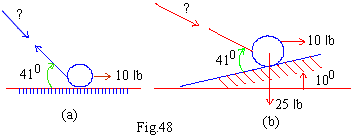In a plane add 120 N Force at 300 and a -100 N force at 900 using the parallelogram method. Ans. R= 11/N , q = 3390 with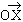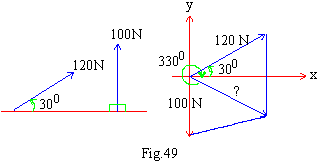Plot the points with the given polar coordinates (i) (-1, 180 ) (ii) (2.3, -400) (iii) (-1, 7p /6 rad) (iv) (5, 5rad) Ans.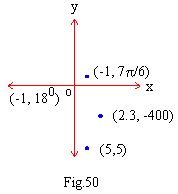Find the set of polar coordinates for the points with given Cartesian coordinates. (i) (-3, 0)    (ii) (2, 2Ö3)    (iii) (5, -12)    (iv) ( 1, -3) Ans. i) (3, 1800)     (ii) (4, 600)   (iii) (13, -67.380   (iv) ( Ö10, -71.570) Convert the equations to polar form   (i) x = 7   (ii) x2+ y2 = 9   (iii) y = 2x   (iv) x2- y2 = 1   (v)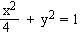Ans.   (i) r cos q =7   (ii) r = 3   (iii) q = tan-1 (2)   (iv) r2 cos 2q = 1   (v) r2 (cos2 q + 4 sin2 q ) = 4 Convert  the  equations  to  Cartesian coordinates       (i) r = 3   (ii) q = 3p/4   (iii) r sin q =7   (iv) r2 cos q = 3 sin q - cos q Ans.    (i)   x2 + y2 = 9     (ii)   y = -x     (iii)   y = 7 (iv)   (x2 + y2 ) x = 3y- x ********* Index Chapter 8
 Search: All Products Books Popular Music Classical Music Video DVD Toys & Games Electronics Software Tools & Hardware Outdoor Living Kitchen & Housewares Camera & Photo Cell Phones Keywords: